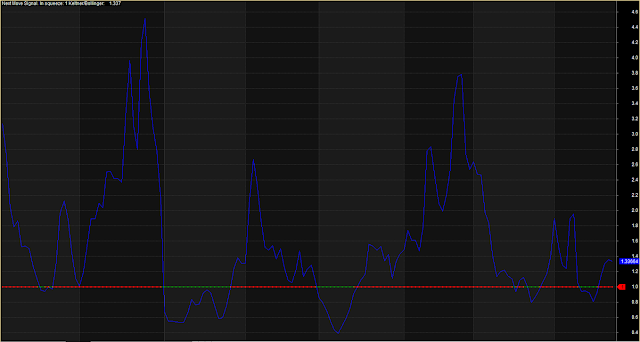.

# Bollinger Band Volatility Indicator - Amibroker AFL CodeBollinger Band Volatility Indicator

```/*
Bollinger bands squeeze.

This is a volatility indicator.
It can be used to determine the periods of extremes of low volatility which usually followed by big moves.
Indicator does not show direction of the trade, only timing.
Some other aspects of technical/fundamental analysis should be employed for direction.

There is a signal line with colored dots. Red dots indicate periods of low volatility (sqeeze).
Green dots indicate periods of high volatility.
Indicator line crosses above and below signal line. Time trades at historical extremes of low volatility.

It can be used for scans, for instance, to find stepper stocks before big moves.

For confirmation look for sqeezes in two different time frames.

*/

Length = 8;
Price = EMA(Close, Length);

// Keltner
kLength = Length;
kN = 1.5;
kATR = ATR(kLength);
kUpper = Price + kN * kATR;
kLower = Price - kN * kATR;

// Bollinger
bbLength = Length;
bbN = 2;
bbStDevValues = StDev(Close, bbLength);
bbUpper = Price + bbN * bbStDevValues;
bbLower = Price - bbN * bbStDevValues;

IsSignal =
bbUpper <= kUpper AND
bbLower >= kLower;

Graph0 = 1;
Graph0Style = styleDots;
Graph0BarColor = IIf(IsSignal, colorRed, colorGreen);

Proportion = (kUpper - kLower) / (bbUpper - bbLower);
Graph1 = Proportion;

Title = "Next Move Signal. In squeeze: " + WriteVal(IsSignal, 1) + " Keltner/Bollinger: " + WriteVal(Proportion);
```